# Fluid Flow

## Bernoulli's Equation in Fluid Mechanics

Fluid is defined as a substance with no fixed shape and yields easily to external pressure. But one of the major features of all fluid is its ability to flow. The fluid includes gas and liquid. This chapter talks about the mechanism behind fluid flow.

FLUID MECHANICS

Fluid mechanics talk about the implementation of the fundamental laws of physics such as that of principles of mechanics and thermodynamics including conservation of mass, conservation of energy and Newton's laws of motion on the behavioural pattern of fluids viz liquid and gas. Fluid mechanics is broadly classified into two categories:

• a) Hydrostatics or fluid statics- revolves around the study of fluid at rest

• b) Hydrodynamics or Fluid Dynamics- the study about the fluid in motion

• This chapter focusses on the fluid dynamics part with special emphasis on fluid behavior under dynamic conditions.

FLUID FLOW

Studying fluid motion is very complicated. But in order to understand the basics of fluid flow certain assumptions have been made about the fluid to simplify the situations. While studying fluid flow, we always consider the fluid to be an ideal one. The assumptions made for an ideal fluid include:

• a) The fluid is incompressible and thus with a constant density

• b) The motion is irrotational that is there is smooth flow(either laminar or streamline), devoid of any turbulence

• c) The fluid is non-viscous without any internal friction

• d) The flow is steady i.e the velocity at each point is constant in time

• The flow of fluid can be broadly classified into two categories :

• 1. Laminar flow

• 2. Turbulent flow

• In a laminar flow, all the particles of a fluid within a layer move at the same rate. On the other hand, the flow is considered turbulent if the critical speed of a fluid is obtained.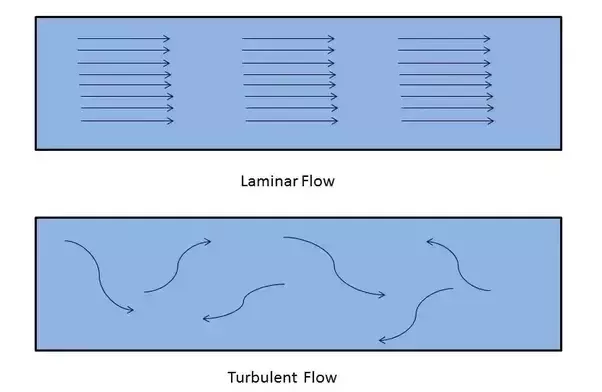Conceptually, fluid flow can be considered analogous to transport process, in which the rate of transfer of matter or energy is dependent on physical factors like the physical properties of the material that has been used (body through which the fluid is moving) and the geometry of that system. Some of the fundamental principles of physics that are considered to study fluid flow are:

Conservation of Mass
Conservation of Energy
Conservation of Linear Momentum

These three conservation laws will form the basis to develop our fundamental understanding of Fluid Flow. Gradually, we will apply these fundamental principles to derive the three major mathematical descriptions of Fluid Flow: the Continuity Equation, Bernoulli’s Equation, and the Momentum Equation. But we need to keep one thing in mind all the time that the fluid considered here is the ideal one. We will try to understand the mechanism of flow of fluids through vessels of various shapes and sizes.

• 1. CONSERVATION OF MASS/CONTINUITY EQUATION

• The basic principle of classical physics is mass is neither created nor destroyed. It is one of the fundamental laws governing fluid motion and it will pave the way for our understanding of fluid dynamics. The figure below shows an infinitesimally small stationary, rectangular control volume. Let’s assume a fluid is moving through this. This type of control volume which has its surface fixed in space is called a Eulerian control volume.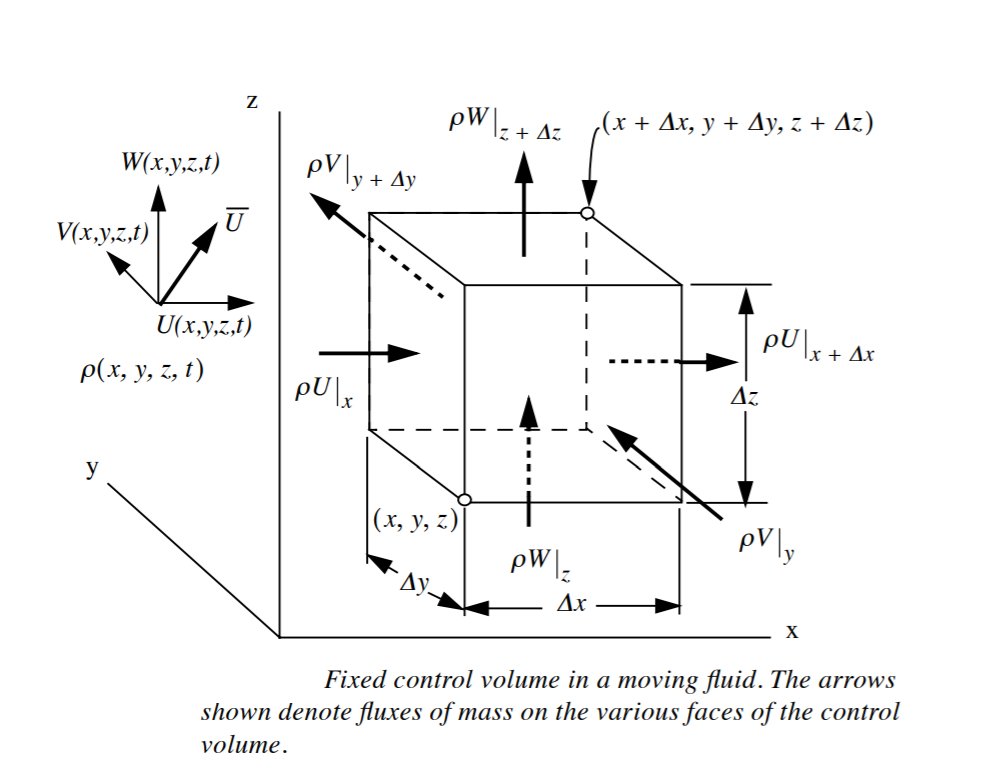The fluid velocity vector has componentsU(vector)=U, V, W in the directions x (vector)=x,y,z and lets the fluid density be ρ. In case of a general, unsteady, compressible flow, all four flow variables may vary depending on position and time. The law of conservation of mass over this control volume can be formulated as: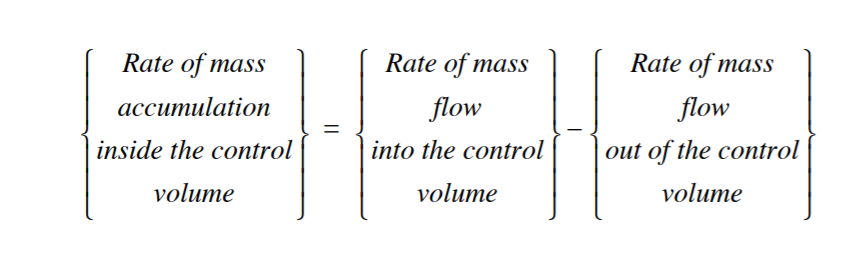• 2. CONSERVATION OF ENERGY

• The concept of conservation of energy during the flow of a fluid can be explained by Bernoulli's equation. We will find that for a fluid (e.g. air) flowing through a pipe with a constriction in it, the fluid pressure is least at the constriction. In terms of the equation of continuity, the fluid pressure falls with the increase in the speed of flow. The reason behind this is explained by the equation. The fluid has different speeds with different kinetic energies at different parts of the tube. The changes in energy are the outcome of work being done on the fluid and the only forces in the tube that work on the fluid are the driving forces related with changes in pressure from place to place.

This equation is concerned about three issues:

• a) Conservation of energy during the flow

• b) A pressurized fluid must contain energy by the virtue of which the work must be done to establish the pressure.

• c) Energy change happens simultaneously with the pressure change in a moving fluid.

•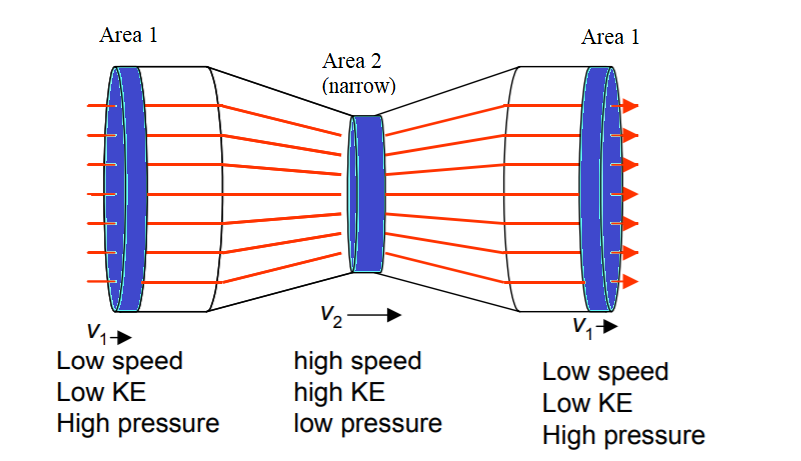Let m be the mass element that moves from (1) to (2)
m = ρ A1 ∆x1 = ρ A2 ∆x2 = ρ ∆V where ∆V = A1 ∆x1 = A2 ∆x2

As per the Equation of continuity, AV = constant
A1 v1 = A2 v2 A1 > A2 ⇒ v1 < v2

Since v1 < v2 the mass element has been accelerated by the net
force
F1 – F2 = p1 A1 – p2 A2

Mass element faces an increase in KE
∆K = ½ m v22 - ½ m v12
= ½ ρ ∆V v22- ½ ρ ∆V v12

The mass element has an increase in GPE
∆U = mg y2 – mg y1 = ρ ∆V g y2 = ρ ∆V g y1

The increase in KE and GPE is generated from the network done on themass element by the forces F1 and F2 (the sample of mass m, inmoving from a higher pressure region to a lowerpressure zone, has some positivework done on it by the surrounding fluid)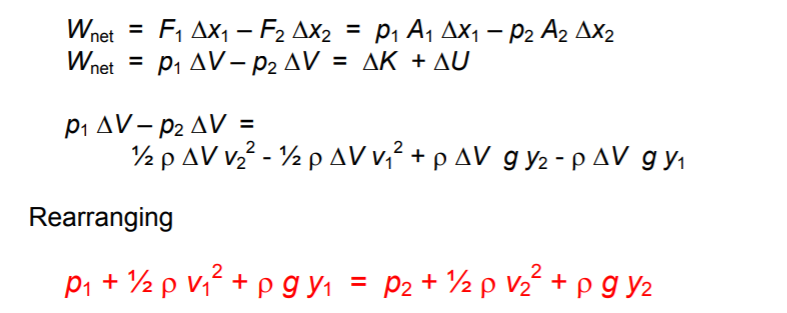We can also conclude that for any point along a flow tube or streamlineThis is what is called the Bernoulli's theorem.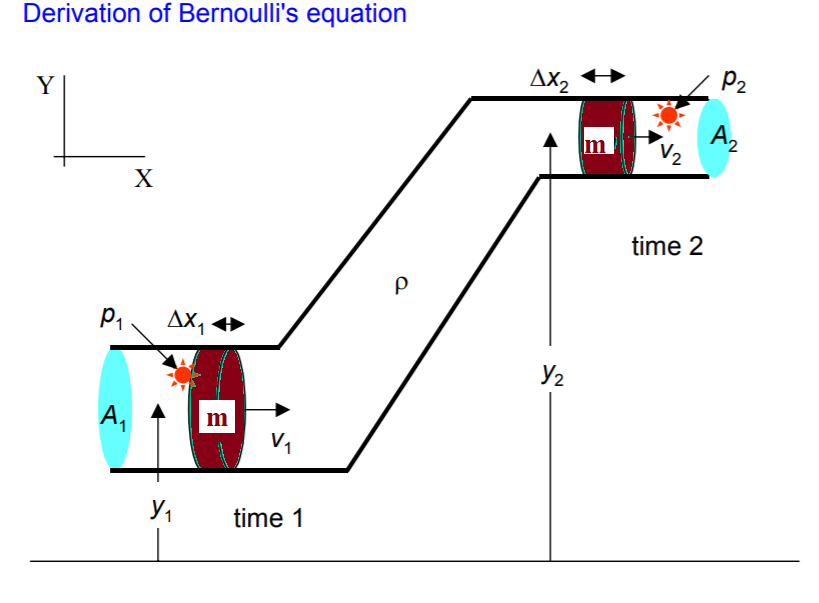Bernoulli's theorem is applicable only to an ideal fluid. Bernoulli's Principle can’t be used when the viscosity is substantial for the fluid in motion. It can be applied to gases as well provided there are only small changes in pressure.

• 3. CONSERVATION OF MOMENTUM/MOMENTUM EQUATION

• From Newton's second law of motion, it has been found that if momentum is gradually decreasing with time, then d (mv) /dt is negative and the force responsible for the change is also negative. This is the force acting on the body resulting in changing momentum. Again, following Newton's third law of motion, there is the equal and opposite force exerted by the body. But if we want to apply this principle of conservation of momentum to fluid flow, we will be struck by two difficulties, as compared to the application of conservation of mass and energy. Firstly, momentum as a conserved quantity is comparatively less familiar to us than mass or energy – we will have slightly more difficult to analyze what exactly is happening. Secondly, and most importantly, both mass and energy are scalar quantities that have magnitude only, unlike momentum which is a vector quantity with both magnitude and direction. Momentum needs to be conserved in a particular direction, so we must be well sensitive to the directions in which various forces are acting and also be careful about the direction of the components moving, and we must (to understand more advanced applications) be able to perform the calculations keeping in mind the several directions simultaneously. With the application of Newton’s Second Law, the product rule gives a form more appropriate for the analysis of fluid flow: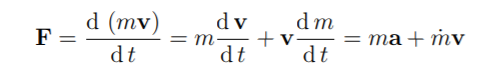where m˙ has been considered as the rate of change of mass (i.e. the rate at which mass comes in or leaves a control volume). Mostly we are focussing on the steady flow, that is it has zero acceleration, and thus we can say, F = ˙mv. As mentioned above, the momentum force exerted by the fluid, in turn, is of the same magnitude, but just in the opposite direction.

Fluid flows has a crucial role to play in a vast variety of phenomena be it natural or manmade systems. In a way or the other, the mechanics and thermodynamics of fluid flow have impacted energy generation, various atmospheric creations,
manufacturing of various vehicles and flights and many more activities. This chapter has attempted to give an overview of fluid dynamics with a special focus on the fundamental principles of fluid mechanics. But one thing should be kept in mind that under a couple of circumstances we have considered a few facts but in the real implementation, there may arise a few issues as there is nothing called ideal fluid.